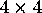Non-Euclidean GeometryNext: Minimal Surfaces Up: Manifolds Previous: Viewing with 4D

### Non-Euclidean Geometry

Mathematicians in the nineteenth century showed that it was possible to create consistent geometries in which Euclid's Parallel Postulate was no longer true- Absence of parallels leads to spherical, or elliptic, geometry; abundance of parallels leads to hyperbolic geometry. By mid-century the English mathematician Arthur Cayley had constructed analytic models of these three geometries that had a common descent from projective geometry, which one may think of as the formalization of the renaissance theory of perspective. Cayley's construction is in fact ideal for programming interactive navigations of nonEuclidean geometries .

Many manifolds are naturally suited for hyperbolic or spherical, rather than Euclidean, geometry. Although the formulas for computing distance and angles in these geometries differ from Euclidean geometry, they can be built into mathematical visualization systems by hand. Translations, rotations, and dot-products for shaders and illumination must also be handled differently in the non-Euclidean geometries. In Figure 8, we see a partial tessellation of regular right-angled dodecahedra in the three built-in Geomview models of hyperbolic 3-space. In the virtual model shown in Figure 8a, the user can fly around to see the tessellation from the insider's point of view. The other two models are from the outsider's point of view, and we can see the position of the insider's camera marked by the blue X.

In the projective (Beltrami-Klein) model of hyperbolic space, shown in Figure 8b, geodesics (the paths of light rays) are Euclidean straight lines, while angles are non-Euclidean in character. In the conformal (Poincaré) model, on the other hand, angles are measured by Euclidean protractors, but light rays travel along circular arcs as in Figure 8c. Figure 9, from the animation Not Knot (Sidebar B), shows us what an insider in an interesting hyperbolic 3-manifold would experience. This 3-manifold is obtained by gluing the faces of a right-angled dodecahedron (an impossibility in Euclidean space) in a way analogous to our imaginary barbershop.

The projective models of non-Euclidean geometry can be represented byreal matrix transformations on homogeneous coordinates that are serendipitously supported by today's computer graphics transformation hardware and software . The conformal model, however, cannot be implemented usingreal matrices, so standard computer graphics hardware does not suffice. Furthermore, the lines between edge endpoints as well as the faces themselves are curved, thus requiring subdivision of polygon edges and faces into small line segments and polygons in order to draw them as curves in computer graphics. Thus, the graphics in the conformal model is considerably slower than in the other two models.

In all cases, however, the standard built-in illumination computations are implicitly Euclidean; correct rendering of surface shading requires custom software shaders that use alternative inner products for computing distances and angles in non-Euclidean geometry. Such shaders have been implemented and shown in  to impose only moderate performance penalties in comparison to the built-in Euclidean ones.

Such interactive tools for the exploration of non-Euclidean spaces show clearly how computer graphics can allow humans to experience worlds that otherwise would not be accessible. Direct manipulation of non-Euclidean rotation and translation allows us to develop an intuition for non-Euclidean behavior that we would be hard put to gain in any other way. For research mathematicians, it provides a microscope for investigating the diverse world of three-dimensional manifolds.

Not all technical problems in geometrical visualization stem from complicated manifolds, dimensions higher than three, or the challenge of representing non-Euclidean geometry. Problems imposing strong geometric constraints on the evolution of surfaces in ordinary 3D space are also of great potential interest. The remaining parts of this section deal with two important domains of this type: minimal surfaces - surfaces that minimize curvatures or energy functionals - and regular homotopies - manifold deformations whose curvatures remain finite at every point. The latter treatment focuses on a particularly fascinating regular homotopy, the sphere eversion.Next: Minimal Surfaces Up: Manifolds Previous: Viewing with 4D

Tamara Munzner
Thu Sep 21 19:17:33 CDT 1995